AP State Syllabus AP Board 7th Class Science Important Questions Chapter 4 Motion and Time

## AP State Syllabus 7th Class Science Important Questions 4th Lesson Motion and Time

### 7th Class Science 4th Lesson Motion and Time Important Questions and Answers

Question 1.
Give few examples of motion you observe in day to day life.

1. Motion is a common experience in our life. We observe birds flying in air, buses, autos, cars, bullock carts, moving on roads, trains on railway tracks and many other objects around us in motion.
2. Apart from observing motions of objects around us, we ourselves experience motion while we are walking, running, playing, riding a bicycle etc.
3. Similarly, we observe many objects like trees, buildings, display boards, electric poles etc., at rest while we walk to school.Question 2.
How do you explain the terms motion and rest? Give an illustrative answer.

1. We know that the position of the car has changed with respect to the tree in ‘2’ seconds. But there is no change in the position of the tree with respect to its surroundings.2. An object is said to be in motion if it changes its position with respect to its surroundings in a given time.
3. An object is said to be at rest if there is no change in its position with respect to its surroundings in a given time.
4. Thus, we can say that the tree is at rest and the car is in motion while we observe them over a period of time (2 seconds in this case).

Question 3.
Explain the terms uniform and non-uniform motions and define them.
Uniform and non-uniform motion:

1. A body is said to be in motion if its position keeps on changing with time (with respect to the observer).
2. But in our daily life we experience certain motions in which, the change in position of objects remains the same for a time interval, in some other motions, the change in position of the object will not be the same for a given time interval.
3. Uniform motion (Definition): If a body covers equal distances in equal intervals of time it is said to be in uniform motion.
4. Non – Uniform motion (Definition): If a body covers unequal distances in equal intervals of time, it is said to be in non – uniform motion.Question 4.
Explain the types of motion with suitable examples.
Types of Motions:

1. When we discuss about motion, it is important to be aware that there exist different types of motions and each type is dependent on a particular situation.
2. Let us consider the following examples.
3. A car travelling along a straight road.
4. Motion of blades of a ceiling fan.
5. Motion of needle in a running sewing machine.
6. Motion of pendulum in an old wall clock.
7. Considering the direction of motion what differences do we notice in above examples?
8. The motions in the above examples are different in terms of direction of motion.
a) Car is moving in same direction along a straight line.b) Blades of ceiling fan rotate about a fixed line in a circular path.c) Needle of sewing machine moves up and down about a fixed point.d) Pendulum of the wall clock oscillates ‘to’ and ‘fro’ about a fixed point.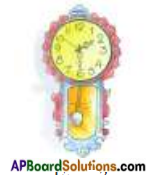9. Based on the path taken by the bodies in motion we classify motion of bodies as,
1) Translatory motion
2) Rotatory motion
3) Oscillatory motion

Question 5.
Define rectilinear motion and curvilinear motion.

1. Rectilinear motion (Definition): If a body in translatory motion moves along a straight line then motion is called rectilinear motion.
2. Curvilinear motion (Definition): If a body in translatory motion moves along a curved path then motion is called curvilinear motion.Question 6.
Identify the types of motion in the examples given below.
We notice everyday some motions which are rectilinear and curvilinear at a time. Some examples of motions are given below; Label them as rectilinear (R) or curvilinear (C) or Rectilinear and curvilinear (RC) motions.
1. Seconds hand of a watch. ( )
2. Movement of a train on tracks. ( )
3. Movement of a tape in a tape recorder. ( )
4. Movement of a needle in a speedometer of car. ( )
5. Movement of a bus on hill station road. ( )
6. Motion of coins on a carrom board. ( )
7. Motion of the ball in pin board. ( )
8. Motion of a mango falling from tree.  ( )
1. Seconds hand of a watch. ( C )
2. Movement of a train on tracks. ( R )
3. Movement of a tape in a tape recorder. ( C )
4. Movement of a needle in a speedometer of car. ( C )
5. Movement of a bus on a hill station road. ( RC )
6. Motion of coins on a carrom board. ( R )
7. Motion of ball in pinboard. ( RC )
8. Motion of a mango falling from tree. ( R )

Question 7.
Explain Rotatory motion with suitable examples.
Observe the following diagrams.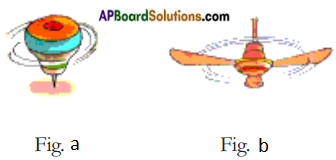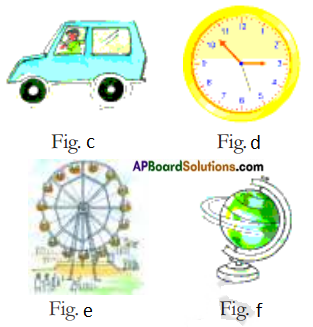1. What similarity do we find in all the motions?
We find all the bodies rotating around an axis.

2. What is the path of motion of each particle of the body that moves?
The path of motion of each particle of the body that moves is circular.

3. Is there any change in the position of a body while it is in motion?
Except car there is no change in the position of the bodies while they are in motion.
The motion of all these bodies is rotatory motion.Question 8.
Explain rotatory motion by taking the blades of a fan moving as an example. Define rotatory motion.

1. While the blade of a fan is moving, the points A, B, and C on the blade move to Av Br Cj first and then move to A2, B, and C2 position,
2. Thus when a fan is in motion, each point on the blade moves in a circular path around the centre of the fan which is fixed.
3. Here, we observe that the position of a fan is not changing.
Only the blades of fan are changing their position continuously and moving in circular path around a fixed point.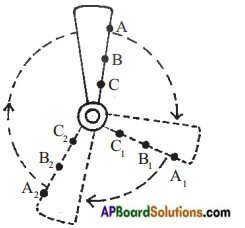4. The imaginary line passing through this fixed point is called axis of rotation.
5. This type of motion is called Rotatory Motion.
6. Rotatory motion (Definition) : Rotatory motion means that, motion of all particles of a moving body follow a circular path with respect to a fixed centre or axis of rotation.

Question 9.
Explain the terms ‘slow’ and ‘fast motions’.

1. We observe many objects in motion in our daily life.
2. In some cases objects move slowly and in other cases they move fast.
3. To know whether the motion is slow or fast

Let us assume that you started to school on a bicycle and your friend started in a bus at the same time from a place.
a) Who reaches the school first? Why?
b) Do you find any difference in the time taken by bicycle and bus to reach the school?
a) My friend reached the school first. Because he started in a bus and I started on a bicycle.
b) Bus reaches the school fast. It means bus took less time to reach the school. Bicycle took more time to reach the school.Question 10.
Observe following pairs of objects that are in motion. Compare their motions and decide which moves slower and which move faster. Mark (S) in relevant box.
a) Whether the motion of a body is slow or fast?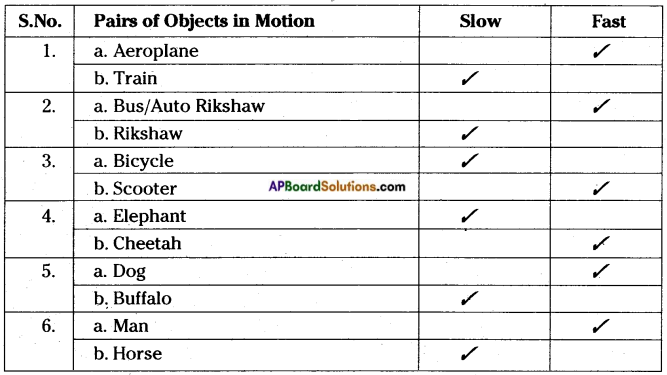a) The body is said to be moving fast if it takes less time to travel the given distance as compared to another body.

Question 11.
Do we need to know about distance covered, time taken by the body in motion to decide whether the motion is slow or fast?
Yes, we need to know about distance covered, time taken by the body in motion, to decide whether the motion is slow or fast.

Question 12.
Priya, Karthik, Divya and Kiran participated in a 100 m. running race. They took 20 s, 22s, 25s and 28s respectively to reach the finishing line. Who do you think ran the fastest and whose running is slowest? Why?

1. Obviously we can see that all of them ran the same distance of 100 m but time taken to cover the distance was different.
2. Priya took the shortest time of 20 seconds which shows that she was the fastest.
3. Kiran took the longest time of 28 seconds which shows that he was the lowest.

Question 13.
Write the units of time.
The basic unit of time is a second(s). Larger units of time are minutes (min) and hours (h).
Table: Units of time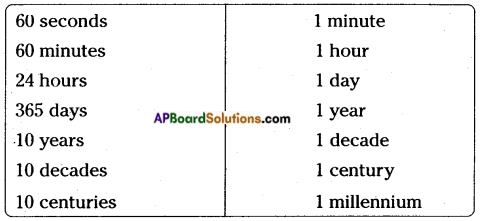Question 14.
What is the need to know the speed? How do we know it?

1. Many objects in the world around us are moving.
2. To compare how fast they are moving, we need to know their speed.
3. You may have observed the speedometer in motor cars which tells us how fast the car is travelling.
4. Usually, the speedometer in a vehicle shows the distance travelled in kilometers and the needle shows the speed of the journey in kilometers per hour.
5. Look at the speedometers of two vehicles shown here and decide which vehicle is slower.
Car – 1 is slower.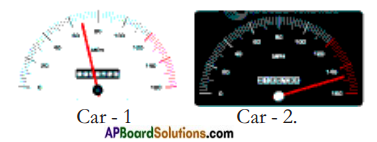Question 15.
How do you calculate the average speed of any object?

1. Average speed = Total distance travelled / Total time taken to travel the distance.
2. Thus we can define speed of an object as the distance travelled by it in a unit of time.

Question 16.
What are the units of speed?
Units of speed.

1. Depending on the need and context, speed is measured in different units.
2. Unit of speed in S.I. system is meter per second (m/s)
3. Another unit commonly used for speed is kilometer per hour (Km/h).
4. The basic unit of speed is m/s.

Question 17.
Observe motion of the car.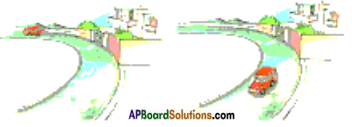1. The distance between the driver and the car changes. ( )
2. The distance between the car and gate of the house is changed. ( )
3. There is no change in the position of gate of the house with respect to its surroundings. ( )
4. There is no change in the position of the car with respect to its surroundings. ( )

1. The distance between the driver and the car does not change.
2. The distance between the car and gate of the house is changed. (Correct statement)
3. There is no change in the position of the gate of the house with respect to its sourroundings (Correct statement)
4. There is change in the position of the car with respect to its surroundings.

Question 18.
Show that 1 km/h = 5/18 m/s.
1 km = 1000 m
and 1 h = 3600 s
1 km / h = 1000 m/3600 s = 5/18 m/sQuestion 19.
Consider a car driven on a road. A person seated beside the driver recorded the distance travelled after every 10 minutes by noting the distance reading in the
speedometer. The distance travelled by the car at different instances of time is as follow.
a) What is the total distance travelled by the car?
b) What is the time taken to travel the distance?

 Time Reading of Distance Covered 0 minute 0 km 10 minute 15 km 20 minute 25 km 30 minute 38 km 40 minute 60 km

c) How do you find the speed of the car?
d) Is the speed of the car uniform throughout the journey?
a) Total distance travelled by the car = 60 km.
b) Time taken = 40 min.
c) To find the speed of the car in the journey we should calculate total distance travelled by the car and the total time taken to cover that distance.
Total distance travelled by the car = 60 km
Time taken = 40 min.
The distance must be either in meters or kilometers and time in seconds or hours. We express the speed either in m/s or in km/h. In this example distance is 60 km and time is 40 min.
1 hour = 60 min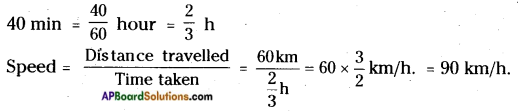Thus, the car travelled with an average speed of 90 km/h.
d) From the table, we notice that the car has covered unequal distances in equal intervals of time (10 min), which shows the speed of the car is not uniform.

Question 20.
The speed of a bus is 72 km/h, whereas the speed of a car is 12.5 m/s. Which vehicle moves faster?
1) The speed of the bus is = 72 km/h
= 72 × $$\frac{5}{18}$$ = 20 m/s
2) The speed of the car is = 12.5 m/s
3) As the speed of the bus is more than the speed of the car, the bus moves faster.
Note: To compare two speeds. They must be expressed in the same units. In the above example, speed of a bus is expressed in km/h and the speed of a car in m/s, tp compare these speeds, one of them must be converted to other.
Speed of a bus = 72 km/h = 20 m/sQuestion 21.
How can you prove that motion is relative to the observer?
Observing motion of the car.
Look at the pictures.

1. Imagine that you sat in the moving car beside the driver.
2. As the car moves, the distance between you and the landmarks like buildings, trees, poles etc., outside the car changes.
3. This change in the scene you view through the window indicates that the car is moving.
4. However your position with respect to the driver of the car remains the same.
5. That is you and the driver of the car are at rest with respect to each other, but both of you are in motion with respect to the surroundings outside the car.
6. A body may be at rest with respect to one set of surroundings and at the same time be in motion with respect to another set of the surroundings.
Thus motion is relative to the observer.

Question 22.
Observe the following pictures. Read the statement below the first picture and write similar statements about other pictures.
a) 1) The man in the boat is moving in respect to the bank of river.2) He is at rest with respect to the boat.

b) 1) The girl on the swing is with respect to the to seat of the swing.A) The girl on the swing is at rest with respect to the seat of the swing.
2) She is with respect to the garden.
A) She is moving with respect to the garden.

c) 1) The girl on the bicycle is in respect to the road.A) The girl on the bicycle is in motion in respect to the road.
2) She is in respect to the bicycle.
A) She is at rest in respect to the bicycle.Question 23.
How are the hands of wall clock and the movement of a butterfly in a garden described?

1. Imagine the movement of hands in a wall clock and the movement of a butterfly in a garden.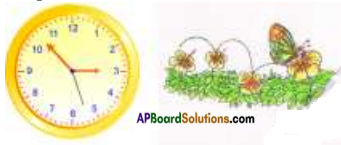2. In these two cases hands of wall clock and the butterfly are in motion.
3. They change their positions with time.

Question 24.
a) What difference do you find in the movement of hands of clock and the body of butterfly?
b) In which case is the change of position with time constant?
a)

1. We observe that in case of the wall clock, Change in position of minute hand is same for every minute.
2. How do we know this?
3. Measuring the angle between two positions of the minute hand is the way.

b) 1) But in the case of the butterfly, the change in its position is not constant while it is flying from one flower to another in the garden.

Question 25.
Observe the following tables, showing distances travelled by two different cars for different instances of time.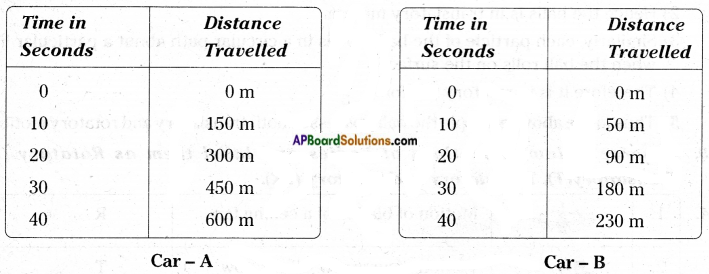1) Which car has travelled equal distances in equal intervals of time?
2) Which car has travelled unequal distances in equal intervals of time?

1. Obviously we notice that for car – A, the change in position in every 10 seconds is 150 m. But for car – B, the change in position is not constant.
2. For 1st 10 seconds, it is 50 m, for 2nd 10 seconds, it is 40 m, for 3rd 10 seconds it is 90 m, and for 4th 10 seconds it is 50 m.
3. Thus motion of car – A is uniform and motion of car – B is non – uniform.Question 26.
Define uniform motion and non- uniform motion.

1. Uniform motion : If a body covers equal distances in equal intervals of time, it is said to be in uniform motion.
2. Non – uniform motion : If a body covers unequal distances in equal intervals of time, it is said to be in non – uniform motion.

Question 27.
Observe the following motions of objects, State whether they are in rotatory motion? Draw a line showing axis of rotation by using pencil.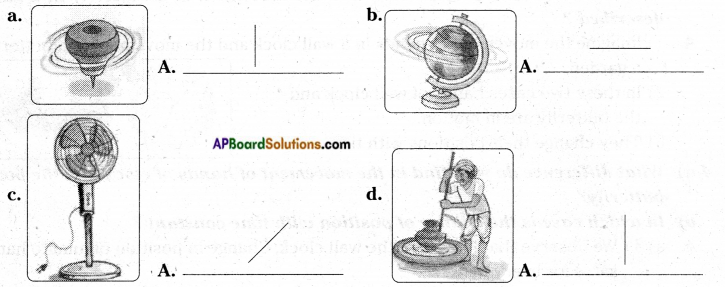Question 28.
Take a table tennis ball, and keep it on the surface of a table. Push the ball with your finger and observe its motion. Is it in Rotatory motion or in Translatory motion?

1. When you push the ball on the surface of a table, it moves from one end to the other end and all the particles of the ball also move along the direction of motion of the ball.2. Hence the balls is in translatory motion.
3. Similarly, each particle of the ball moves in a circular path about a particular line, when the ball rolls on the surface.
4. Therefore it is in rotatory motion.
5. Thus in the above example the ball possesses both translatory and rotatory motions.Question 29.
Observe following motions of bodies and label them as Rotatory (R), Translatory(T), Translatory and Rotatory (TR).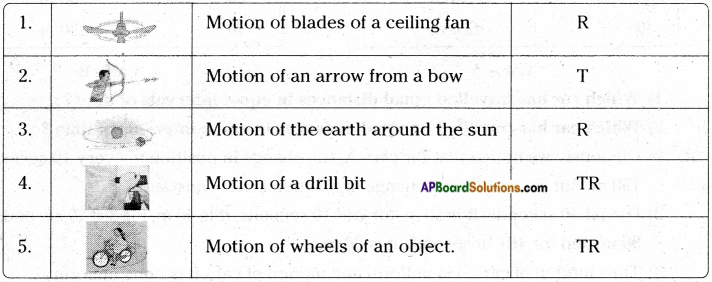Question 30.
Take a cell phone. Go to ‘setting’ and open ‘stop clock’ option. If you are not able to do this take help from your friend or teacher. Measure the time taken for the events mentioned in the table.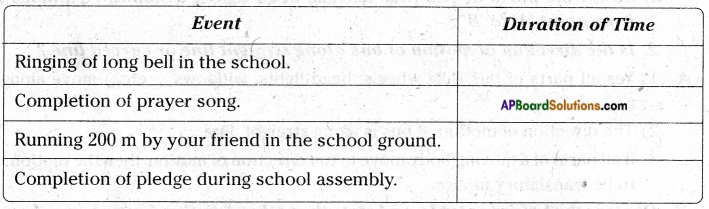Which event has taken more time to complete? Think! How much time does it take to sing the national anthem?
The student is advised to do this activity in the school and fill up the columns.

Question 31.
Write the motion of different parts of a bicycle while it is in motion.
a) The wheel: Rotatory and Translatory motion.
b) The cycle chain: Rotatory motion.
c) The pedal with its arm: Rotatory.
d) The movement of feet pedaling Rotatory.
e) The rider along with the bicycle; Translatory.

Question 32.
Identifying Uniform and Non – Uniform motion.
Identify uniform and non uniform motions among the following examples and mark Uniform as (U) and Non-Uniform as (NU).
1. Movement of hands of a clock. ( U )
2. A boy cycling in a crowded place. ( NU )
3. Movement of a housefly. ( NU )
4. The fan in an air cooler running at fixed speed. ( U )
5. A train entering into a railway station,. ( NU )
6. Kite in the air ( NU )
7. Rotation of the earth. ( U )Question 33.
Look at the following pictures.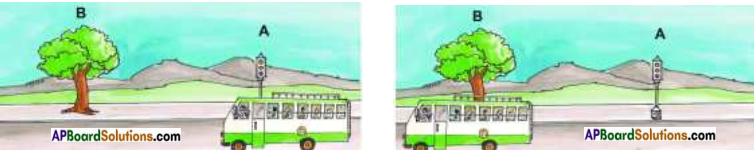The bus moved from point ‘A’ to ‘B’ in a certain interval of time.
Think and answer the following questions:
1. Do all the parts of bus (like wheels, head lights, windows etc., move along from point A’ to B’?
2. Is the direction of motion of bus along straight line or curved line?

1. Yes all parts of bus (like wheels, head lights, windows … etc.,) move along from point A to B.
2. The direction of motion of bus is along straight line.
3. If all parts of a moving body move in the direction of motion then the motion is said to be translatory motion.

Question 34.
Observe the following table, and state the paths of motion for each case by putting (✓) mark in the relevant column.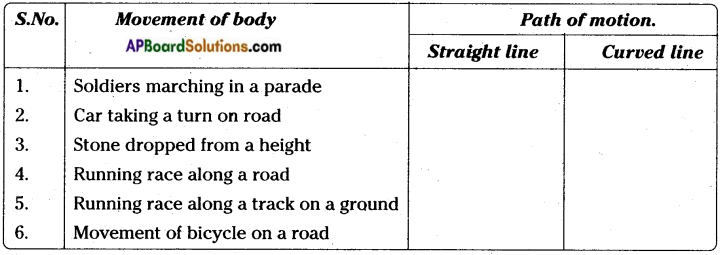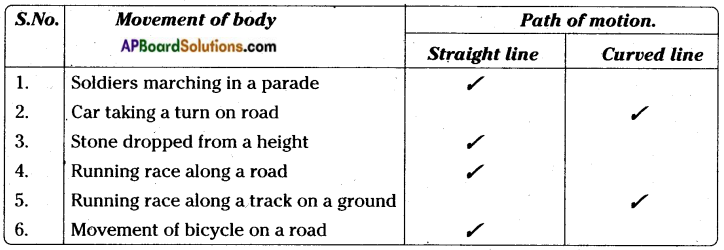Question 35.
Identify oscillatory motion among the following and put (✓) mark in the brackets given.
A spinning top (- )
Bullet from a gun (-)
Typewriter key (✓)
Motion of a potter’s wheel. (-)
Motion of a vibrating sitar string. (✓)
Motion of a car taking a turn while moving. (-)
Ringing of a bell (✓)
A bouncing belli (✓)

Question 36.
Observe the following pictures and answer the following questions.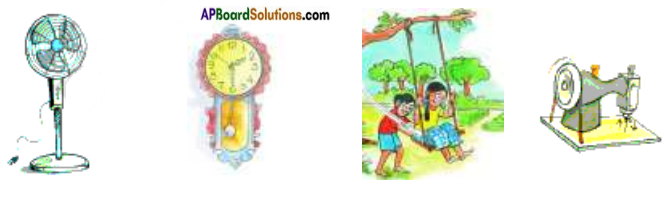1. What similarity do you find in the motions of the above given situations?
2. Are the objects in motion following the same path again and again?
3. Is the direction of motion constant?
4. What type of motion is this?
5. Define oscillatory motion.

1. If we critically examine the above mentioned motions, we understand that in each case the objects move backwards and forwards or upward and downward, on the either side of a fixed point or a line.
2. Yes. For example, the girl on a swing moves backwards and forwards, on either side of the rest position of the swing.
3. Similarly in other cases also the body is in ‘to’ and ‘fro’ motion along the same path of motion.
4. This type of motion is called oscillatory motion.
5. Oscillatory motion: (Definition)
The ‘to’ and ‘fro’ motion of an object about a fixed point always following the same path is called oscillatory motion.Question 37.
How do you communicate the meaning of the terms ‘faster’ and ‘slower’ with the help of situation showing drawings?
We understand that the distance travelled by an object in a given interval of time can help us to decide which one is faster and which one is slower.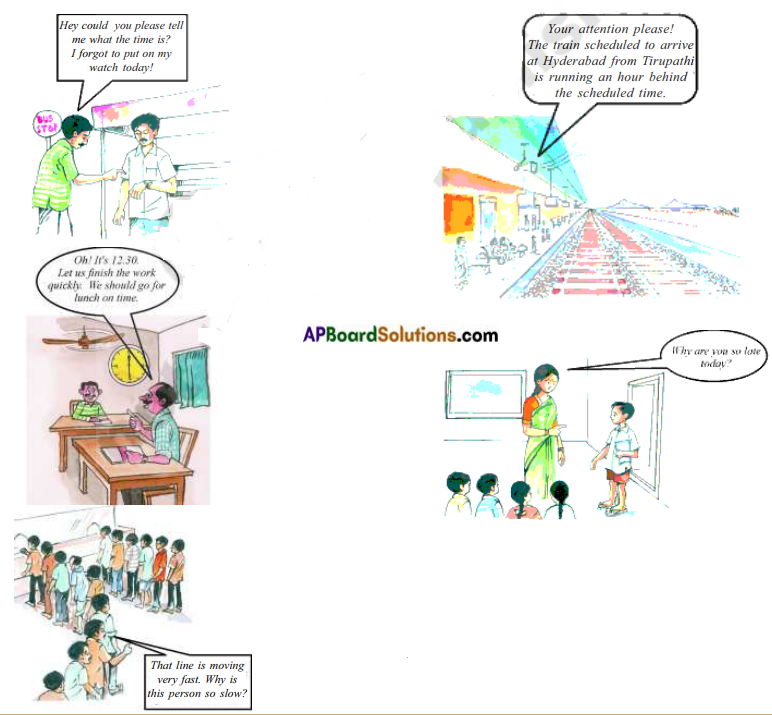1. We use the word time very often in our daily life. Look at the following examples.
2. If we observe the above examples, we use the word ‘time’ for different purposes.
3. In some situations, without using the word ‘time’, we express the duration of time like ‘so late’, so early’ etc.

Question 38.
How do we measure or estimate time? Explain with an example.

1. Ravi and Sathish started for school at 8.00 a.m. from their houses which are side by side. Ravi started on a bicycle and Sathish by walk.
• Who will reach the school first? How much earlier will he be compared to the other?
• How do you measure ‘early’ or ‘late’ arrival at school?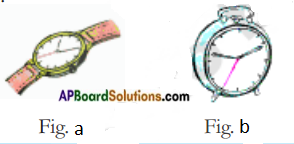2. You can easily estimate that Ravi reaches the school earlier than Sathish.
3. But to answer the question of how early Ravi reached, we need to measure the times taken by both Ravi and Sathish and find the difference of time between both the cases.
4. For this we need time measuring instruments like watches, clocks etc.
5. Nowadays, we use different instruments like electronic clocks, digital clocks, quartz clocks etc. to measure time.
6. A few decades ago people used pendulum clocks that have now become rare.Question 39.
What are the different units for measuring time?
Like minutes and hours, week, fortnight, month, season, ayanam are also units for measuring time.

Question 40.
How is the measurement of time done?

1. Stop Clocks are used to measure time accurately.
2. We have seen stop clocks in the laboratory.3. We use stop clocks in the laboratory to measure short intervals of time like time taken for completion of chemical reaction, time taken by the pendulum for one oscillation etc.
4. Nowadays we find stop clocks almost in all cell phones.
5. In our daily life stop clocks help measure pulse rate etc.
6. Apart from this, they also help us to accurately measure the times in running races, swimming races, etc.

Question 41.
Travel on a motor vehicle with your father to market or any other place and observe the changes in speedometer reading.
Note the exact time when you started from home and observe the speedometer reading carefully and note it and the corresponding time in the table. Some examples are given in the table.

 Speedometer Reading Corresponding Time (Speed) (Time) 0 Started at home 9-10 a.m. 20 9.15 a.m. 30 9.20 a.m.

a) Does the speed of the vehicle remain the same throughout the journey?
b) If not, what can you say about the speed of the vehicle during the journey?

1. We experience in our everyday life that most of the objects in motion do not have the same speed throughout the journey.
2. To express the speed of the object, we consider its average speed.
a) The speed of the vehicle is not the same throughout the journey.
b) If such is the case, we consider its average speed.

Question 42.
We can classify motion based on the path taken by the bodies during motion. What are translatory motion and rotatory motion?
Translatory motion: If all parts of a moving body move in the direction of motion, then the motion is said to be translatory motion.
Rotatory motion: Movement of any object about an axis.Question 43.
i) How many types of motions are there and what are they?
ii) Read the table and identify the motion.
If the motion is Rotatory, put (R), Translatory (T), both Rotation and Translatory (RT), Oscillatory (O).

 1. Motion of ceiling fan 2. Motion of needle in the sewing machine 3. Motion of Earth around the sun 4. Drilling bit while drilling a wood plank 5. Motion of wheels in moving cycle 6. Motion of moving top (Bongaram) 7. Motion of the wire in the harp (Veena) 8. Raising the rubber ball from the floor

a) There are 3 types of motions.
They are: 1) Translatory motion; 2) Rotatory motion and 3) Oscillatory motion.

b)

 1. Motion of ceiling fan R 2. Motion of needle in the sewing machine O 3. Motion of Earth around the sun TR 4. Drilling bit while drilling a wood plank TR 5. Motion of wheels in moving cycle TR 6. Motion of moving top (Bongaram) R 7. Motion of the wire in the harp (Veena) T 8. Raising the rubber ball from the floor O

Question 43.
Why do you think the objects like trees, buildings, electric poles, etc. appear to be moving when you are sitting inside a moving bus or train?

1. An object may be in the state of movement and at the state of rest at the same time.
2. It happens when the objects compared with different surroundings.Question 44.
Write the motion of different parts of a bicycle while it is in motion.
i) The wheel
ii) The cycle chain
iii) The pedal with its arm
iv) The movement of the rider along with the bicycle